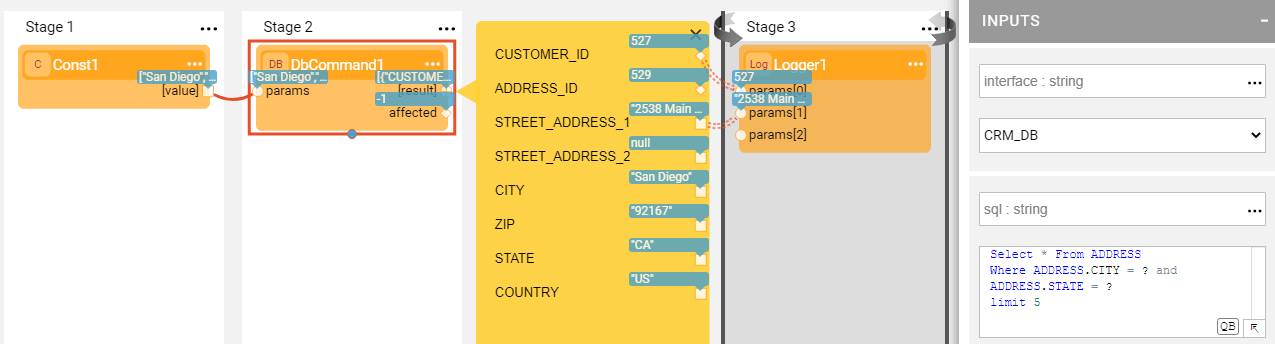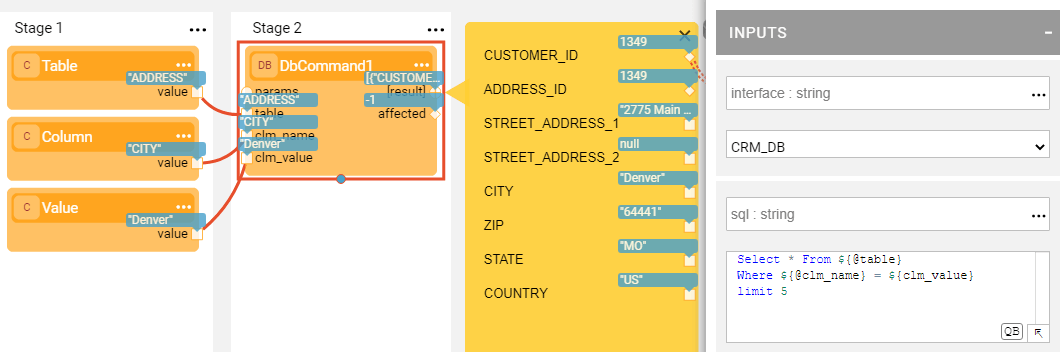XDB Command Actors

Broadway has a category of db Actors that are useful for performing DB commands and actions like creating a new table, loading data or fetching it and executing other DB commands. These Actors are:

• DbLoad Actor, loads data into a database using an INSERT, UPDATE or UPSERT command.
• DbCommand Actor, performs database commands on a DB interface. It has two extensions:
• DbFetchField Actor, returns the first field of the first row or null if this is empty.
• DbFetchFirstRow Actor, returns the first row or an empty row if there is no result.
• DbCreateTable Actor, creates a new database table.
• DbCassandraCreateTable Actor, creates a new Cassandra table.
• DbDelete Actor, deletes data from a database using DELETE based on keys.

Each Actor in the db category requires an interface input argument that can be defined either as a reference to the Fabric DB Interface or as a JDBC URL.

The schema, table, fields and sql input arguments of db Actors are case-insensitive.

If the DB command executed by the db Actor fails, the actual SQL statement is sent to the log file. For example:

Caused by: java.sql.SQLException: [SQLITE_CONSTRAINT]  Abort due to constraint violation
(UNIQUE constraint failed: CONTRACT_COPY.CONTRACT_ID)
INSERT INTO "main"."CONTRACT_COPY" ("CUSTOMER_ID","CONTRACT_ID","CONTRACT_REF_ID") VALUES (?,?,?)

How Can I Load the Data?

To load the data using the DbLoad Actor, populate the Actor's input arguments as follows:

• command, select INSERT, UPDATE or UPSERT from the dropdown list.
• When performing an UPDATE or an UPSERT command, you can set ignoreNull input argument to true. In this mode, the SQL statement will not contain fields that have null values.
• schema, table, either type it in or click the DB button to select it from the DB Table Selection popup.
• fields, keys, if a table has been selected, the fields and keys are automatically populated from the DB schema. If not, type in the field names.

Using DbCommand Actor

Another way to load the data in a Broadway flow is by using the DbCommand Actor and writing the SQL INSERT statement in the sql input argument. The values to be populated in the table can be taken from the input arguments using the parameters. For example:

INSERT INTO DATA (TEXT) VALUES (\${text} )

Where \${text} is replaced with the value of the text input argument in the prepared statement.

Parameters and Non-Prepared Statements Support

The DbCommand Actor’s sql input argument includes an SQL statement which is executed by the Actor. The SQL statement can be created dynamically using prepared and non-prepared statement parameters.

The syntax is:

? - for ordered parameters.

\${param_value} - for named parameters.

\${@param} - for non-prepared statement parameters.

The values of named parameters are taken from the Actor's input arguments or from the params input argument and only if it is a map. For ordered parameters, the params input argument should be an array or a single value (not a map).

For example, the SQL statement:

Select * From CASES where STATUS = 'Open'

Can be written in the following way:

Select * From \${@table} where \${@column} = \${case_sts}

The values for the table, column and case_sts input arguments are passed to the Actor where they are translated into an SQL statement. When the Actor is called several times, if the resulting SQL is the same as in the previous run, the prepared statement is not recalculated.

Examples

The db-commands.flow example shows how the DbCommand Actor can be used to perform various DB actions, including:

• Creating a table using the SQL provided in the sql input argument.
• Inserting the data into the table using the sql input argument with named parameters marked as \${text}. The parameter's values are populated from the params input argument.
• Selecting the data from the table using the sql input argument with an ordered parameter marked as ?.

Click Actions > Examples in the Main menu to open the db-commands.flow example.Example of Passing Parameters to SQL

The following example shows how to execute a SELECT statement which includes a WHERE clause using ordered params:The parameters for the WHERE clause are transferred using the Const Actor's [values] object connected to the params input argument of the DbCommand Actor.

[
"San Diego",
"CA"
]

The following example shows how a SELECT statement is executed using named params:When the above query is written in the sql input parameter, a new city_name input argument is added to the DbCommand Actor and the parameter is transferred to it.

Example of Non-Prepared Statement

The following example shows the SQL statement which includes parameters to populate the table and column name:The new input arguments table, clm_name and clm_value are added to the SourceDbQuery Actor and are populated by 'cases', 'status' and 'Open' values respectively.

In this example, table and clm_name are non-prepared statement parameters while clm_value is a prepared statement named parameter.

DB Command Actors

Broadway has a category of db Actors that are useful for performing DB commands and actions like creating a new table, loading data or fetching it and executing other DB commands. These Actors are:

• DbLoad Actor, loads data into a database using an INSERT, UPDATE or UPSERT command.
• DbCommand Actor, performs database commands on a DB interface. It has two extensions:
• DbFetchField Actor, returns the first field of the first row or null if this is empty.
• DbFetchFirstRow Actor, returns the first row or an empty row if there is no result.
• DbCreateTable Actor, creates a new database table.
• DbCassandraCreateTable Actor, creates a new Cassandra table.
• DbDelete Actor, deletes data from a database using DELETE based on keys.

Each Actor in the db category requires an interface input argument that can be defined either as a reference to the Fabric DB Interface or as a JDBC URL.

The schema, table, fields and sql input arguments of db Actors are case-insensitive.

If the DB command executed by the db Actor fails, the actual SQL statement is sent to the log file. For example:

Caused by: java.sql.SQLException: [SQLITE_CONSTRAINT]  Abort due to constraint violation
(UNIQUE constraint failed: CONTRACT_COPY.CONTRACT_ID)
INSERT INTO "main"."CONTRACT_COPY" ("CUSTOMER_ID","CONTRACT_ID","CONTRACT_REF_ID") VALUES (?,?,?)

How Can I Load the Data?

To load the data using the DbLoad Actor, populate the Actor's input arguments as follows:

• command, select INSERT, UPDATE or UPSERT from the dropdown list.
• When performing an UPDATE or an UPSERT command, you can set ignoreNull input argument to true. In this mode, the SQL statement will not contain fields that have null values.
• schema, table, either type it in or click the DB button to select it from the DB Table Selection popup.
• fields, keys, if a table has been selected, the fields and keys are automatically populated from the DB schema. If not, type in the field names.

Using DbCommand Actor

Another way to load the data in a Broadway flow is by using the DbCommand Actor and writing the SQL INSERT statement in the sql input argument. The values to be populated in the table can be taken from the input arguments using the parameters. For example:

INSERT INTO DATA (TEXT) VALUES (\${text} )

Where \${text} is replaced with the value of the text input argument in the prepared statement.

Parameters and Non-Prepared Statements Support

The DbCommand Actor’s sql input argument includes an SQL statement which is executed by the Actor. The SQL statement can be created dynamically using prepared and non-prepared statement parameters.

The syntax is:

? - for ordered parameters.

\${param_value} - for named parameters.

\${@param} - for non-prepared statement parameters.

The values of named parameters are taken from the Actor's input arguments or from the params input argument and only if it is a map. For ordered parameters, the params input argument should be an array or a single value (not a map).

For example, the SQL statement:

Select * From CASES where STATUS = 'Open'

Can be written in the following way:

Select * From \${@table} where \${@column} = \${case_sts}

The values for the table, column and case_sts input arguments are passed to the Actor where they are translated into an SQL statement. When the Actor is called several times, if the resulting SQL is the same as in the previous run, the prepared statement is not recalculated.

Examples

The db-commands.flow example shows how the DbCommand Actor can be used to perform various DB actions, including:

• Creating a table using the SQL provided in the sql input argument.
• Inserting the data into the table using the sql input argument with named parameters marked as \${text}. The parameter's values are populated from the params input argument.
• Selecting the data from the table using the sql input argument with an ordered parameter marked as ?.

Click Actions > Examples in the Main menu to open the db-commands.flow example.Example of Passing Parameters to SQL

The following example shows how to execute a SELECT statement which includes a WHERE clause using ordered params:The parameters for the WHERE clause are transferred using the Const Actor's [values] object connected to the params input argument of the DbCommand Actor.

[
"San Diego",
"CA"
]

The following example shows how a SELECT statement is executed using named params:When the above query is written in the sql input parameter, a new city_name input argument is added to the DbCommand Actor and the parameter is transferred to it.

Example of Non-Prepared Statement

The following example shows the SQL statement which includes parameters to populate the table and column name:The new input arguments table, clm_name and clm_value are added to the SourceDbQuery Actor and are populated by 'cases', 'status' and 'Open' values respectively.

In this example, table and clm_name are non-prepared statement parameters while clm_value is a prepared statement named parameter.